## BACKGROUND

Coronaviruses are a group of viruses that cause serious respiratory infections of humans and animals. Covid-19, the recent contagious pandemic disease which shook the whole world is caused by one such Coronavirus, the severe acute respiratory syndrome coronavirus 2 (SARS-CoV-2). The disease is characterized by cold, cough, fever and in the severe form presents as pneumonia of lungs.

## MACHINE LEARNING IN DIAGNOSIS OF COVID-19

The foremost step in fighting the disease is to identify the infected patients early enough so as to provide them adequate support and to further prevent the spread. Currently, the gold standard test for diagnosis of Covid-19 is the Reverse transcriptase polymerase chain reaction (RT-PCR). Nevertheless, there are several challenges associated, such as the need of dedicated instruments, need of experienced personnel, the possibility of false negative results and takes at least two days to complete. Thus, there is an alarming need to look for alternative methods for rapid and accurate diagnosis of Covid-19.

In this context, chest radiological imaging in the form of X-rays or Computed tomography (CT), might be helpful. Although, chest CT provides efficient and sensitive diagnosis of Covid-19, it is expensive, uses high levels of radiation and cannot be employed for screening purposes. On the other hand, X-ray is less sensitive than CT, however it is cheaper, faster and best alternative for routine diagnosis. X-rays for Covid-19 are characterized with the presence of multiple, patchy, sub-segmental, or segmental ground glass opacities in the lungs. These subtle patterns can only be identified and interpreted by experienced radiologists. Considering the dearth of trained radiologists and enormous number of suspected individuals, there is an urgent need to develop automatic methods that can identify such abnormalities and assist in the rapid diagnosis of Covid-19. Machine learning or Deep learning algorithms such as Convolutional Neural Networks (CNN), can serve as powerful tools for solving such problems.

## WHAT IS CNN MODEL?

CNN is a class of deep learning algorithm, widely used for image classification. Compared to other algorithms, it requires much less pre-processing and can achieve better results with enough training. It is analogous to the neuron operating characteristic of human brain.

## ARCHITECTURE OF CNN MODEL

CNN is made up of an input layer, multiple hidden layers and an output layer. The hidden layers include convolutional layers, pooling layers, fully connected layers and activation function layers. The convolution and pooling layers extract features from the input image while the fully connected layer maps the extracted feature into final output, such as classification.

## OBJECTIVE

Given the vast application of CNN for image classification, a deep learning model can be built to extract features from the chest x-ray images and eventually aid in detection of Covid-19.

The model for the current study has been built using Python, Keras and Tensorflow. Entire coding has been carried out on Google’s Colab environment with Tesla GPU.

## DATA STRUCTURE

Data for the study has been downloaded into google drive as “Data” from the open web source:

Disclaimer: The below link corresponds to version 1, published on 09-06-2020. With the availability of data, the versions get updated. Hence, the users are advised to check the data, the version and modify the code accordingly.

data.mendeley.com

The database consists of 488 augmented chest x-ray images, of which 107, 233 and 148 belong to Covid-19 infected, healthy/normal and pneumonia infected individuals, respectively. The corresponding labels are, ‘CV’, ’N’ and ‘P’. The data has been split using split folders in the ratio of 70:30 as train and validation, respectively.

### We will be using Python for implementation

```import splitfolders
splitfolders.ratio("/content/drive/MyDrive/Data", output="output", seed=1337, ratio=(0.7, 0.3),group_prefix=None) ```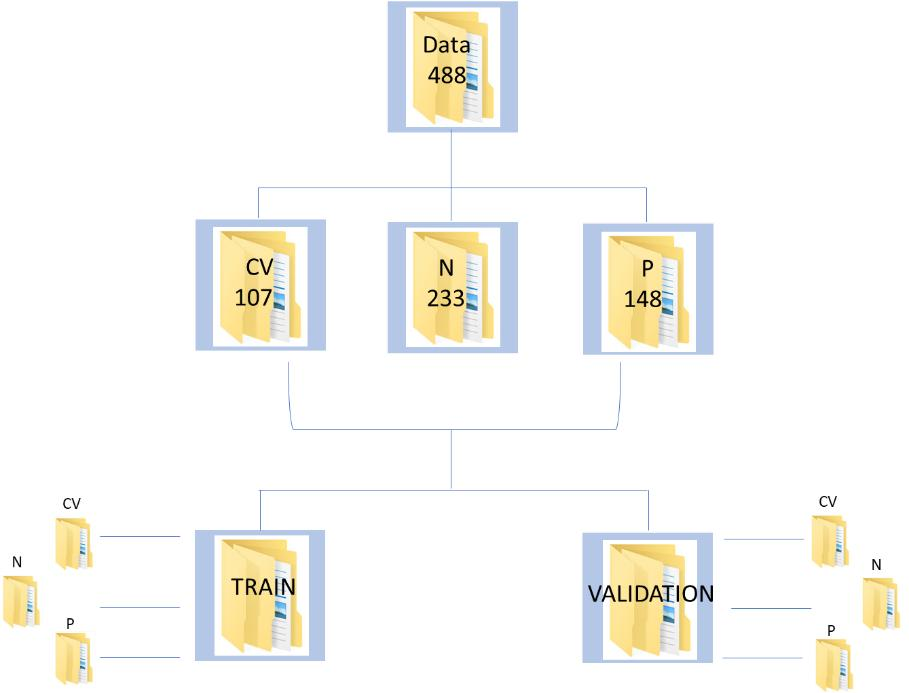Figure 1 : Data Schema for the CNN implementation on Covid Chest XRay Data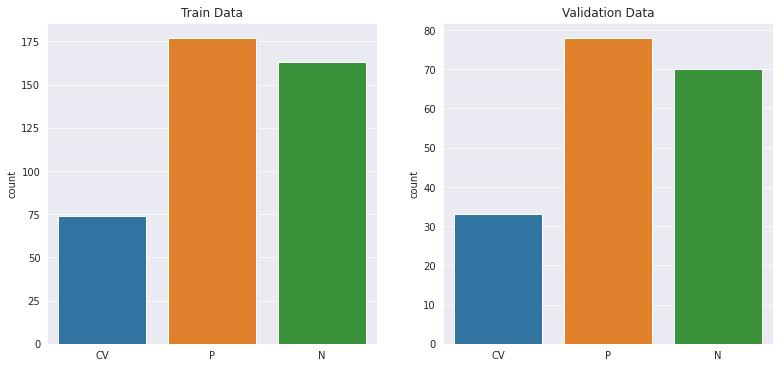Figure 2 : Distribution of image samples in the training and validation data sets

## VISUALIZING FEW IMAGES

```img_path="/content/drive/MyDrive/Data/CV/covid-19-pneumonia-53.jpg"
cv2_imshow(img)

img_path="/content/drive/MyDrive/Data/N/IM-0033-0001-0001.jpeg"
cv2_imshow(img)

img_path="/content/drive/MyDrive/Data/P/person28_virus_63.jpeg"
cv2_imshow(img)
```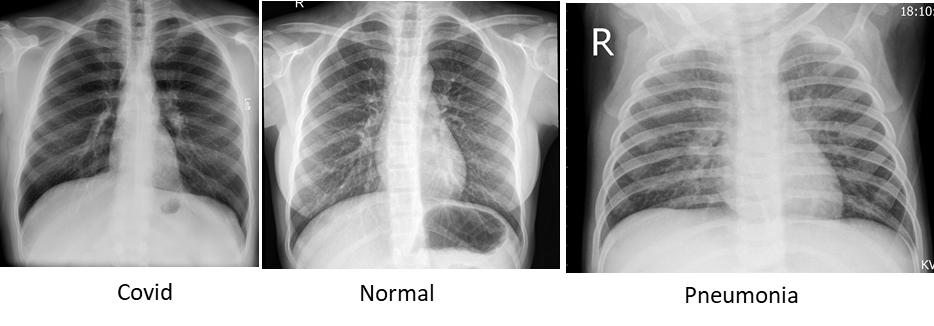Figure 3 : Sample Images of Chest X-Ray with Covid, Normal and Pneumonia dipictions

## IMPORTING NECESSARY LIBRARIES

```from keras_preprocessing.image import ImageDataGenerator
from sklearn.metrics import classification_report, confusion_matrix
from mlxtend.plotting import plot_confusion_matrix
from google.colab.patches import cv2_imshow
from keras_preprocessing import image
from sklearn.metrics import roc_auc_score
from sklearn.model_selection import KFold
from sklearn.model_selection import cross_validate
from sklearn.model_selection import RepeatedKFold, cross_val_score
from keras.wrappers.scikit_learn import KerasClassifier
from keras.preprocessing import image
from keras.layers import MaxPooling2D
from keras.models import Sequential
from keras.models import load_model
from keras.layers import Conv2D
from keras.layers import Flatten
from keras.layers import Dense
import warnings
warnings.filterwarnings('ignore')
from google.colab.patches import cv2_imshow
import cv2
from IPython.display import Image
import matplotlib.pyplot as plt
import tensorflow
import seaborn as sns
from numpy.random import seed
seed(1)
from tensorflow import keras
import keras_preprocessing
from tensorflow import keras
import matplotlib.cm as cm
from numpy.random import seed
from skimage import transform
from PIL import Image
import tensorflow as tf
import numpy as np
import pandas as pd
import h5py
import os
import PIL
import cv2```

## DATA AUGMENTATION

Training a CNN on limited data results in overfitting and subsequently the model might not generalize well on unseen data. To prevent this, data augmentation techniques such as zoom in and out, cropping, horizontal flipping etc, which can increase the diversity of data can be applied.

```train_dir="/content/output/train"
train_datagen=ImageDataGenerator(
rescale=1./255,
shear_range=0.2,
zoom_range=0.2,
horizontal_flip=True,
)

val_dir="/content/output/val"
val_datagen=ImageDataGenerator(
rescale=1./255)

train_generator=train_datagen.flow_from_directory(train_dir,
target_size=(224,224),class_mode='categorical',batch_size=32)

valid_generator=val_datagen.flow_from_directory(val_dir,
target_size=(224,224),class_mode='categorical',batch_size=32,shuffle=False)```

## DEFINING THE MODEL

A model is defined with four convolutional layers, max-pooling layers, a dropout layer to avoid overfitting and the activation function, Relu, which adds non-linearity to the data is included in the hidden layers. Since the task is a multi-label classification, final output layer includes Softmax function, which predicts the probability of individual classes.

```unit=[]
for x in range (0,len(train_generator.class_indices)):
unit.append(x)

units=x+1
model1 =tf.keras.models.Sequential([
tf.keras.layers.Conv2D(64,(3,3),activation='relu',input_shape=(224,224,3)),
tf.keras.layers.MaxPooling2D(2,2),
tf.keras.layers.Conv2D(64,(3,3),activation='relu'),
tf.keras.layers.MaxPooling2D(2,2),
tf.keras.layers.Conv2D(128,(3,3),activation='relu'),
tf.keras.layers.MaxPooling2D(2,2),
tf.keras.layers.Conv2D(64,(3,3),activation='relu'),
tf.keras.layers.MaxPooling2D(2,2),
tf.keras.layers.Flatten(),
tf.keras.layers.Dropout(0.5),
tf.keras.layers.Dense(512,activation='relu'),
tf.keras.layers.Dense(units=units,activation='softmax')
])```

## COMPILING AND TRAINING THE MODEL

The model is next compiled with Adam optimizer, categorical cross entropy as the loss function and trained for 10 epochs. The evaluation metric used is ‘Accuracy’, defined as the percentage of correct predictions made by the model.

```model1.compile(loss='categorical_crossentropy',optimizer='adam',metrics=['accuracy'])
history=model1.fit(train_generator,epochs=10,validation_data=valid_generator)
model1.summary()```

## MODEL EVALUATION

The model is then evaluated by plotting the loss function and the accuracy of train versus the validation data.

```loss_train = history.history['loss']
loss_val = history.history['val_loss']
acc_train = history.history['accuracy']
acc_val = history.history['val_accuracy']

epochs = range(1,11)

plt.figure(figsize=(15, 15))
plt.subplot(2, 2, 1)
plt.plot(epochs, loss_train, 'g', label='Training loss')
plt.plot(epochs, loss_val, 'b', label='Validation loss')
plt.legend(loc='lower left')
plt.title('Training and Validation loss')
plt.xlabel('Epochs')
plt.ylabel('Loss')

plt.subplot(2, 2, 2)
plt.plot(epochs, acc_train, 'g', label='Training accuracy')
plt.plot(epochs, acc_val, 'b', label='Validation accuracy')
plt.legend(loc='lower right')
plt.title('Training and Validation accuracy')
plt.xlabel('Epochs')
plt.ylabel('Accuracy')

plt.show()```

At the end of 10 epochs, the model achieves a training accuracy of 0.78 and validation accuracy of 0.86. The training and validation loss was found to be 0.45 and 0.37, respectively. You can observe the parameters w.r.t the epochs in figure 4 below.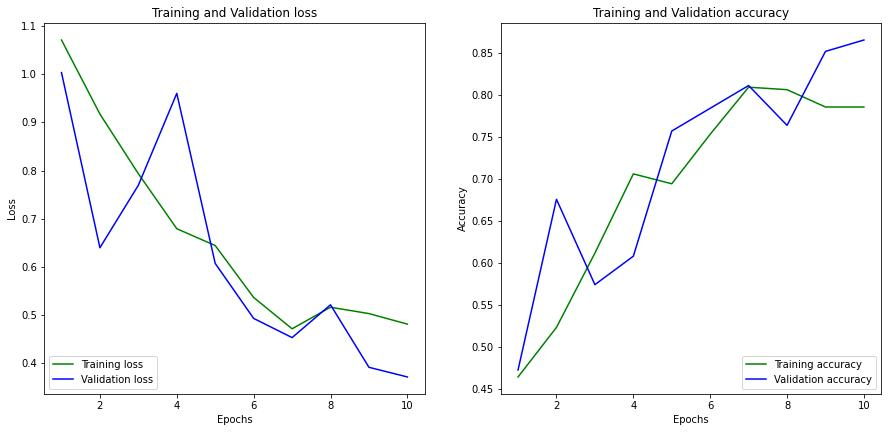Figure 4: Graph of Training Loss Vs Validation Loss along with Training Accuracy Vs Validation Accuracy

## PREDICTIONS, CONFUSION MATRIX, CLASSIFICATION REPORT AND ROC_AUC SCORE

Predictions are then made on validation data and performance of the model is evaluated in terms of accuracy, precision and recall through confusion matrix. ROC_AUC score is another metric used, which measures the ability of the model to distinguish between classes.

```Y_pred = model1.predict_generator(valid_generator)
y_pred = np.argmax(Y_pred, axis=1)
conf=confusion_matrix(valid_generator.classes,y_pred)
classnames = ['CV (Class 0)', 'N (Class 1)', 'P (Class 2)']
plt.title('Confusion matrix of Validation data')
sns.heatmap(conf, xticklabels=classnames, yticklabels=classnames, annot=True,cmap="YlGnBu")
plt.xlabel('Predicted')
plt.ylabel('Actual')
plt.show()
print(classification_report(valid_generator.classes, y_pred, target_names=classnames))

y_pred2=model1.predict(valid_generator)
roc_auc=roc_auc_score(valid_generator.classes,y_pred2,multi_class='ovo')
print('ROC_AUC score on Validation data is:',roc_auc)```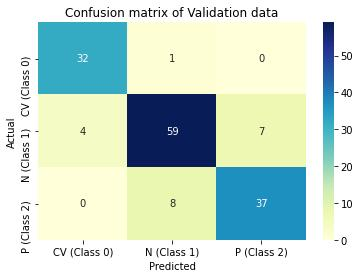Figure 5 : Graphical Illustration of CNN models confusion matrix on training and validation data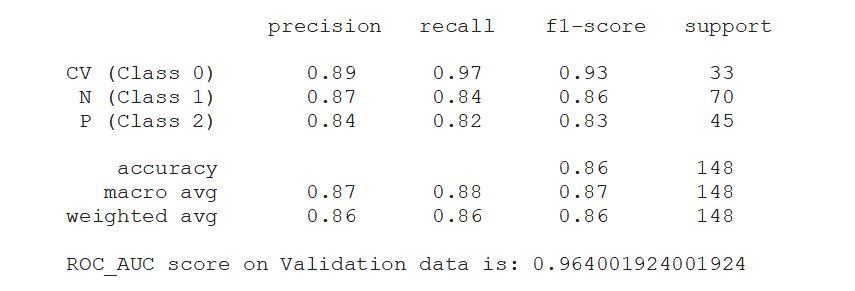## GRADIENT WEIGHTED CLASS ACTIVATION MAP (Grad-cam)

Grad-cam is a popular technique of making class-specific heatmap by highlighting the regions of input image that the CNN has considered relevant to perform predictions. It helps to visualize the output of a CNN.

```last_conv_layer_name = "conv2d_3"
model1_layer_names = [
'max_pooling2d',
'max_pooling2d_1',
'max_pooling2d_2',
'max_pooling2d_3',
'flatten',
'dropout',
'dense',
'dense_1'
]
class_index=0

img_path="/content/drive/MyDrive/Data/P/person28_virus_62.jpeg"
cv2_imshow(img)```
```
np_image = Image.open(file)
np_image = np.array(np_image).astype('float32')/255
np_image = transform.resize(np_image, (224, 224, 3))
np_image = np.expand_dims(np_image, axis=0)
return np_image

myfile="/content/drive/MyDrive/Data/P/person28_virus_62.jpeg"
print(model1.predict(image))

[model1.get_layer(last_conv_layer_name).output, model1.output])

with tf.GradientTape() as tape:
convol_outputs, predictions = gradient_model(image)
loss = predictions[:, class_index]

output = convol_outputs
last_conv_layer_output = output.numpy()

for i in range(pool_grads.shape[-1]):
last_conv_layer_output[:, :, i] *= pool_grads[i]
heat_map = np.mean(last_conv_layer_output, axis=-1)
heat_map = np.maximum(heat_map, 0) / np.max(heat_map)
plt.matshow(heat_map)
plt.show()

img_path="/content/drive/MyDrive/Data/P/person28_virus_62.jpeg"
img = keras.preprocessing.image.img_to_array(img)

heat_map = np.uint8(255 * heat_map)
jet = cm.get_cmap("jet")
jet_colors = jet(np.arange(256))[:, :3]
jet_heat_map = jet_colors[heat_map]
jet_heat_map = keras.preprocessing.image.array_to_img(jet_heat_map)
jet_heat_map = jet_heat_map.resize((img.shape, img.shape))
jet_heat_map = keras.preprocessing.image.img_to_array(jet_heat_map)

# Superimposing the heatmap on original image
superimposed = jet_heat_map * 0.4 + img
superimposed = keras.preprocessing.image.array_to_img(superimposed)
superimposed```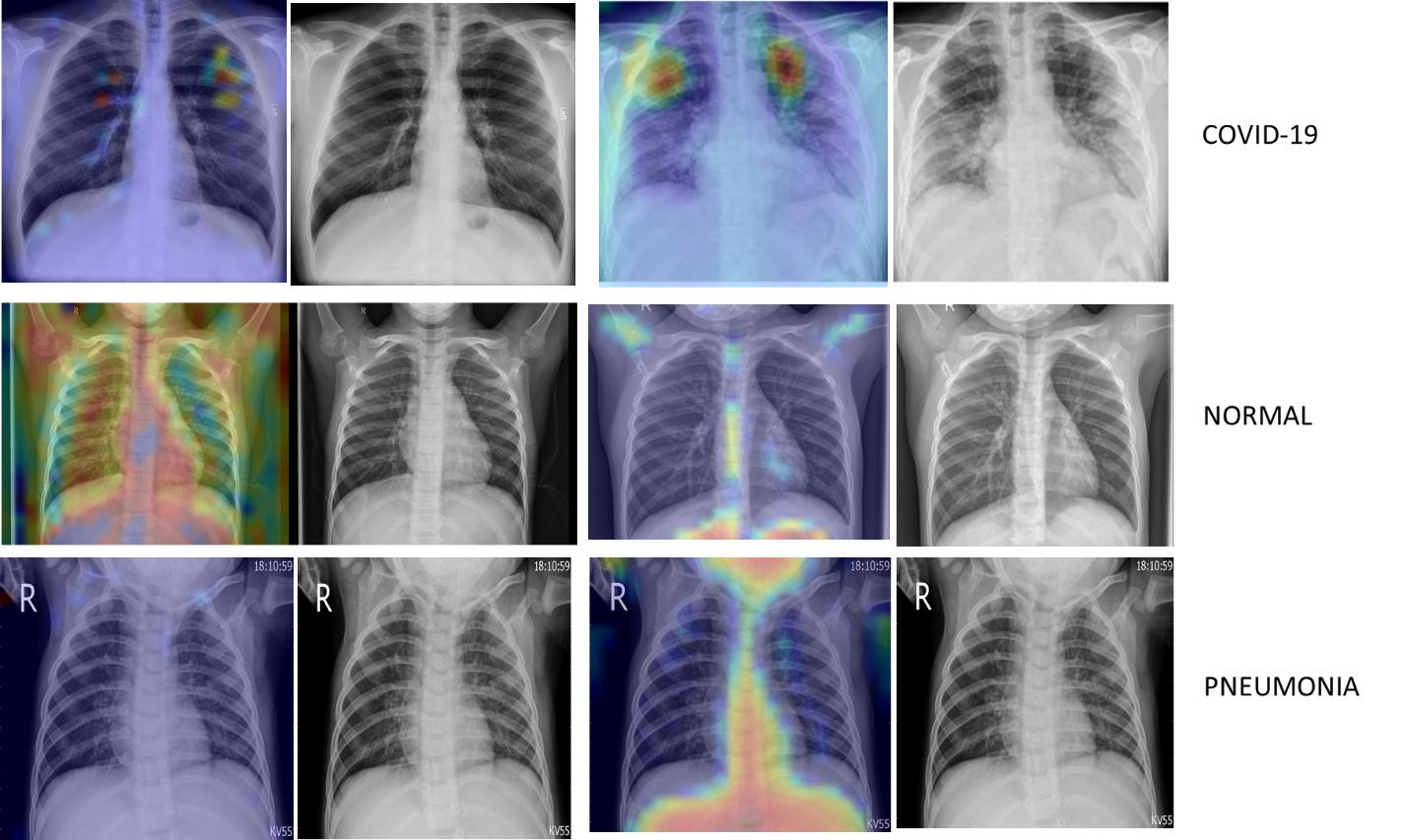Figure 6 : Class Specific Heat Map using Grad-cam

## K-FOLD CROSS VALIDATION

Cross-validation is an important technique to assess the performance of a model. In k-fold cross validation, the data is split into k folds and each fold is used as a test set by the model to make predictions.

```labels = ['CV', 'N','P']
img_size = 224

def fetch_data(dir):
data = []
for label in labels:
path = os.path.join(dir, label)
class_num = labels.index(label)
for img in os.listdir(path):
try:
img_arr = cv2.imread(os.path.join(path, img))[...,::-1]
resized_arr = cv2.resize(img_arr, (img_size, img_size))
data.append([resized_arr, class_num])
except Exception as e:
print(e)
return np.array(data)

data=fetch_data("/content/drive/MyDrive/Data")
a = []
b = []

for feature, label in data:
a.append(feature)
b.append(label)

# Data Normalization
a = np.array(x) / 255
a.reshape(-1, img_size, img_size, 1)

def get_model():
model =tf.keras.models.Sequential([
tf.keras.layers.Conv2D(64,(3,3),activation='relu',input_shape=(224,224,3)),
tf.keras.layers.MaxPooling2D(2,2),
tf.keras.layers.Conv2D(64,(3,3),activation='relu'),
tf.keras.layers.MaxPooling2D(2,2),
tf.keras.layers.Conv2D(128,(3,3),activation='relu'),
tf.keras.layers.MaxPooling2D(2,2),
tf.keras.layers.Conv2D(64,(3,3),activation='relu'),
tf.keras.layers.MaxPooling2D(2,2),
tf.keras.layers.Flatten(),
tf.keras.layers.Dropout(0.5),
tf.keras.layers.Dense(512,activation='relu'),
tf.keras.layers.Dense(3,activation='softmax')
])

return(model)

Classifier= KerasClassifier(build_fn=get_model, epochs=10, batch_size=10, verbose=0)
kfold= KFold(n_splits=6)
precision= cross_val_score(Classifier, a, b, scoring='precision_micro',cv=kfold)
print('Precision is:',precision)
accuracy= cross_val_score(Classifier, a, b, scoring='accuracy',cv=kfold)
print('Accuracy is:',accuracy)
recall= cross_val_score(Classifier, a, b, scoring='recall_micro',cv=kfold)
print('Recall is:',recall)
f1= cross_val_score(estimator, x, y, scoring='f1_micro',cv=kfold)
print('F1 score is:',f1)```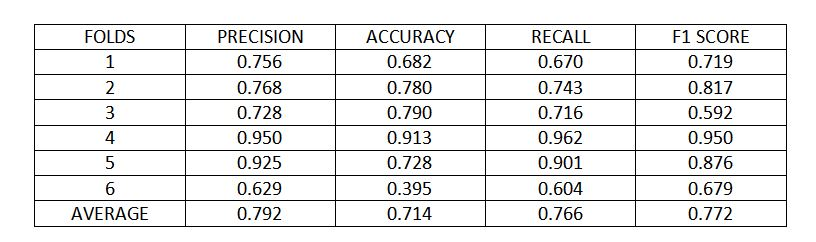Figure 7 : K fold cross validation metrics

Using 6 folds cross validation, the model achieved 79% precision, 71% accuracy, 76% recall and 77% score, on average over different folds.

## CONCLUSION

In conclusion, the proposed model looks promising in identifying and distinguishing Covid-19 from the other two classes of healthy and pneumonia. However, the major limitation of the study is the limited sample size, addressing this problem along with tuning of certain other hyper parameters can further enhance the performance of the model.